A.4 Density of States

When going from small electron systems, such as atoms and molecules, to large electron systems, solids for instance, the number of available electron states reaches high values so that it is best expressed in terms of a density per energy and volume. In solid state theory the assumption of periodic boundary conditions is frequently employed and delivers simple first-order approximation of the density of states (DOS). However, in a few cases, such as tunneling, DOS decomposed in one energy component perpendicular (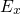) and one parallel () to a certain plain is required. The corresponding derivation will be outlined in the following.

In each direction with periodic boundary conditions, each quantum number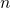is directly related to one wavevector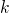: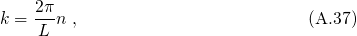If the length of the periodicity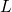is increased, the single quantum states narrows in thespace andbecomes an continuous quantity. Then the summation over the single states can be replaced by an integral.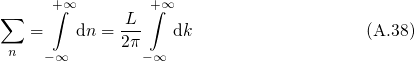Making use of the relation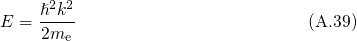one obtains the number of states in the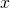-direction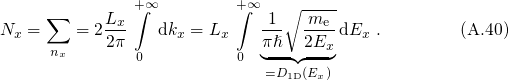For periodic boundaries in two dimensions, an integral transformation to polar coordinates is carried out in order to obtain the number of states in the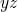-plane.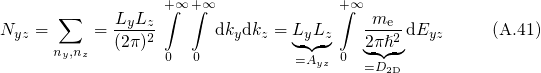with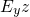being the energy in the-plane. Combining both solutions yields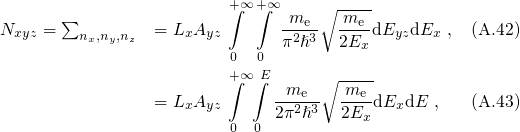where an integral transformation from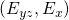to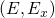with the constraint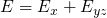is performed. The split one/two dimensional DOS is defined as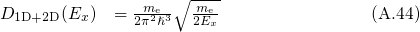while the commonly known three dimensional DOS for a free electron gas reads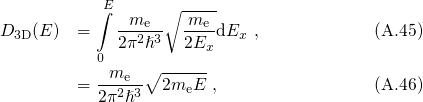which is usually found in textbooks .

For the case that the electrons are confined in the-direction, the number of states are counted in the following way: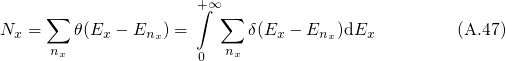Here,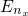denotes the quasi-bound states with the quantum number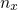. In order to refer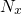to the unit volume, one must introduce the the square of the wavefunction.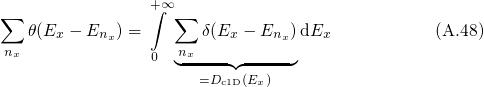When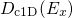is multiplied with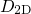and the same integral transformation as for the derivation of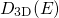is performed, one obtains the DOS for an one-dimensionally confined electronic system: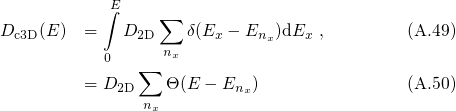Note that in this derivation the spin degeneracy introducing a factor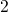has been neglected.